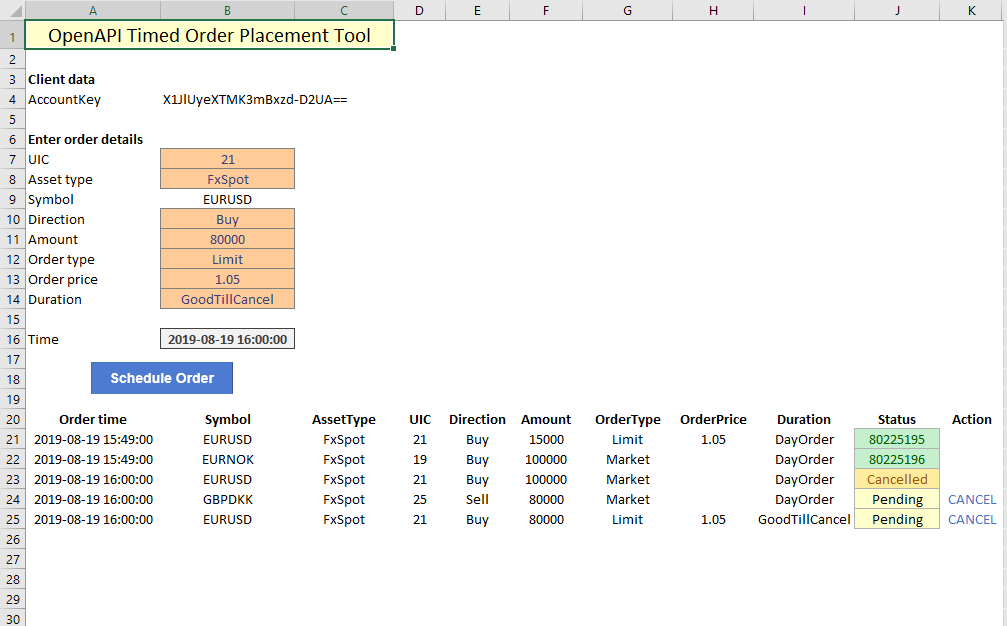OpenAPI for Excel

For this tutorial, we are taking a closer look at Excel VBA's scheduling functionality to create a list of order that will be routed at some future time. This is useful for scheduling or planning order placement along some predefined timings.

As in earlier tutorials, we start with the basics for order placement: gathering the order details and listing out orders that are currently pending. The date and time specified under the "Time" field controls when an order is sent off. Multiple orders can be sent at the same time, but (like with the bulk trader tool before), they will be spaced out to prevent rate limiting.

The internal logic hinges on a simple procedure to determine whether an order needs to be sent off:

1. When a new order is added to the list, the time at which is to be executed is scheduled using the Application.OnTime function in VBA.
2. When OnTime calls the order placer, it takes the current time and checks which of the orders should be sent by comparing each order's planned execution time. If the planned time is the current time (or in the past), the tool triggers order placement.
3. The status is updated when the order is placed, which takes it out of rotation for future runs of the order placer.
4. To prevent rate limiting, the tool pauses for 500 milliseconds.## VBA implementation

The ScheduleOrder routine is fairly basic. It finds the next empty line in the order list, it checks to make sure you are not trying to place an order in the past, and it loads the order details onto the schedule. Next, it calls the Application.OnTime routine, which is where the logic discussed above is contained.

```Sub ScheduleOrder()

Dim nextline As Integer
nextline = 21

Do While Range("A" & nextline) <> "" ' find next blank line
nextline = nextline + 1
Loop

If Now > [ordertime].Value Then
MsgBox "Please pick a time in the future!", , "Error in scheduled time."
Exit Sub
End If

' load order parameters into blank line
Range("A" & nextline & ":J" & nextline) = Array( _
[ordertime].Value, _
[symb].Text, _
[atype], _
[uic], _
[Direction], _
[amount], _
[otype], _
[oprice], _
[odur], _
"Pending")

Range("J" & nextline).Style = "Note"
Range("J" & nextline).HorizontalAlignment = xlCenter

Application.OnTime [ordertime], "ThisWorkbook.SendOrders"

End Sub```

The SendOrders routine handles order placement like we've seen before in earlier tutorials, specifically the bulk order placement tool. The important part of the logic is contained right at the top. For as long as there is data in the order schedule list, if the timing in column A is equal to the current time, or a time in the past, and the order is in 'Pending' state, the OpenAPI call is triggered.

This logic prevents cancelled orders (state: cancelled) from being executed, and it will not touch 'future' orders. Because this is a generic function, it can run at any moment and will only execute orders up until that moment. For each time Application.OnTime calls this routine, it will cycle through the list and find the exact order it was meant to place.

```Sub SendOrders()
' Loops through the list of scheduled orders and finds any that should be send

Dim nextline As Integer
Dim body As String
Dim order As String
nextline = 21

Do While Range("A" & nextline) <> "" And Now >= Range("A" & nextline).Value ' find next order to send by time
If Range("J" & nextline).Text = "Pending" Then ' if the order is pending to be sent
Debug.Print ("sending order on line " & nextline)

body = "{" & _
"'AccountKey':'" & [acckey] & "', " & _
"'Amount':" & Range("F" & nextline).Value & ", " & _
"'AssetType':'" & Range("C" & nextline).Text & "', " & _
"'BuySell':'" & Range("E" & nextline).Text & "', " & _
"'Uic':" & Range("D" & nextline).Value & ", " & _
"'OrderType':'" & Range("G" & nextline).Text & "', " & _
"'OrderPrice':" & Range("H" & nextline).Value & ", " & _
"'OrderDuration':{'DurationType':'" & Range("I" & nextline).Text & "'}, " & _
"'ManualOrder':true" & _
"}"

Debug.Print (body)

order = Application.Run("OpenAPIPost", "trade/v2/orders", body)

If InStr(3, order, "OrderId") Then
Range("J" & nextline) = Mid(order, 13, 8)
Range("J" & nextline).Style = "Good"
Range("J" & nextline).HorizontalAlignment = xlCenter
Else
Range("J" & nextline) = "Error occured"
Range("J" & nextline).Style = "Bad"
Range("J" & nextline).HorizontalAlignment = xlCenter
End If

Sleep (500)

End If

nextline = nextline + 1
Loop

End Sub```